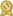# Igor Z.

## specializes in Highly Qualified Math, Physics, Stats Tutor: Understanding is the key! ·

"Private Math, Physics, Statistics Tutoring on ALL LEVELS!

Promise to show “beyond the cookbook” solutions for any problem --- real understanding is the key!

While “cookbook methods” will work on some sample problems, they will not work on real tests!

My real specialty is Advanced Statistics, but I also specialize in pretty much all other areas in Math, Physics and Statistics more...

"Private Math, Physics, Statistics Tutoring on ALL LEVELS!

Promise to show “beyond the cookbook” solutions for any problem --- real understanding is the key!

While “cookbook methods” will work on some sample problems, they will not work on real tests!

My real specialty is Advanced Statistics, but I also specialize in pretty much all other areas in Math, Physics and Statistics: Algebra, Calculus, Geometry as well as more advanced areas (like Linear Algebra, Multivariate Calculus, etc.).

In Physics, my specialties include all the areas of AP Physics: Projectile Motion, Newton's Laws, Conservation of Energy, Electricity and Magnetism, Oscillations Equations, Waves, Optics, etc.

In Statistics, we cover Discrete and Continuous distribution, Sampling Distributions (including Confidence Intervals), Null Hypothesis testing, etc.

Here is a nice example of a problem which does not require any advanced math, but still just as interesting:

find: S = 1+ 2 + 3 + … 998 + 999 + 1000?

Solution is replace all the additions with ONE MULTIPLICATION!

My approach is to ensure that the student really understands the subject matter. It's always better to go slow so that the student is not overwhelmed by too much new material. It's also great when a particular problem can be analyzed in a number of different ways which will "converge" on the same answer. In addition, I like to assign some of my own homework which will hopefully make student think about some of the concepts in a "deeper way". I also really like and try to emulate the approach of Gilbert Strang from MIT (great professor of Linear Algebra). His approach is to ask the right questions and guide students towards the logical answers." less...

# Details

 fee: \$120 (for 60 min) travel distance: 15 miles meeting type: in-person (not online)

# Contact

 website: on file

# Education and Qualifications

 certified:City College of New York, undergrad

University of Chicago, Masters

Columbia College Columbia University, Masters

# Subjects Tutored

Algebra 1 - Algebra 2 - Calculus - Microsoft Excel - Geometry - Physics - Prealgebra - Precalculus - Trigonometry - Statistics - SAT Math - SQL - Probability - ACT Math - Differential Equations - Actuarial Science - Linear Algebra - MATLAB